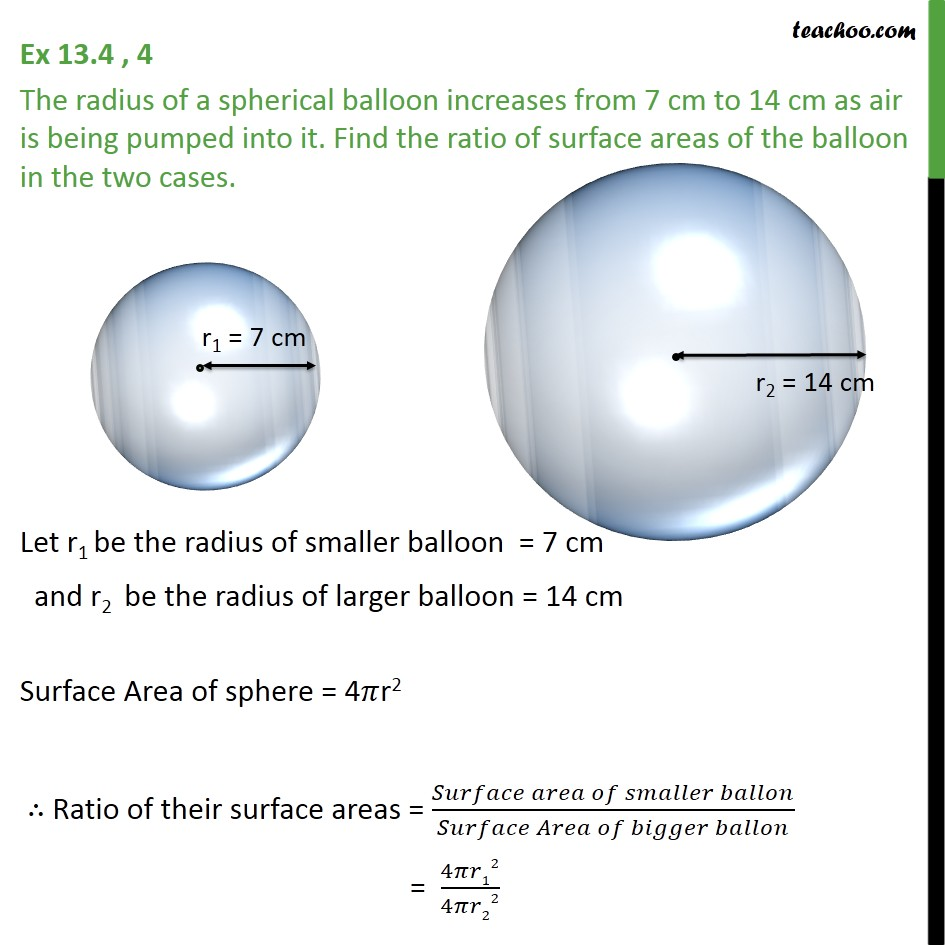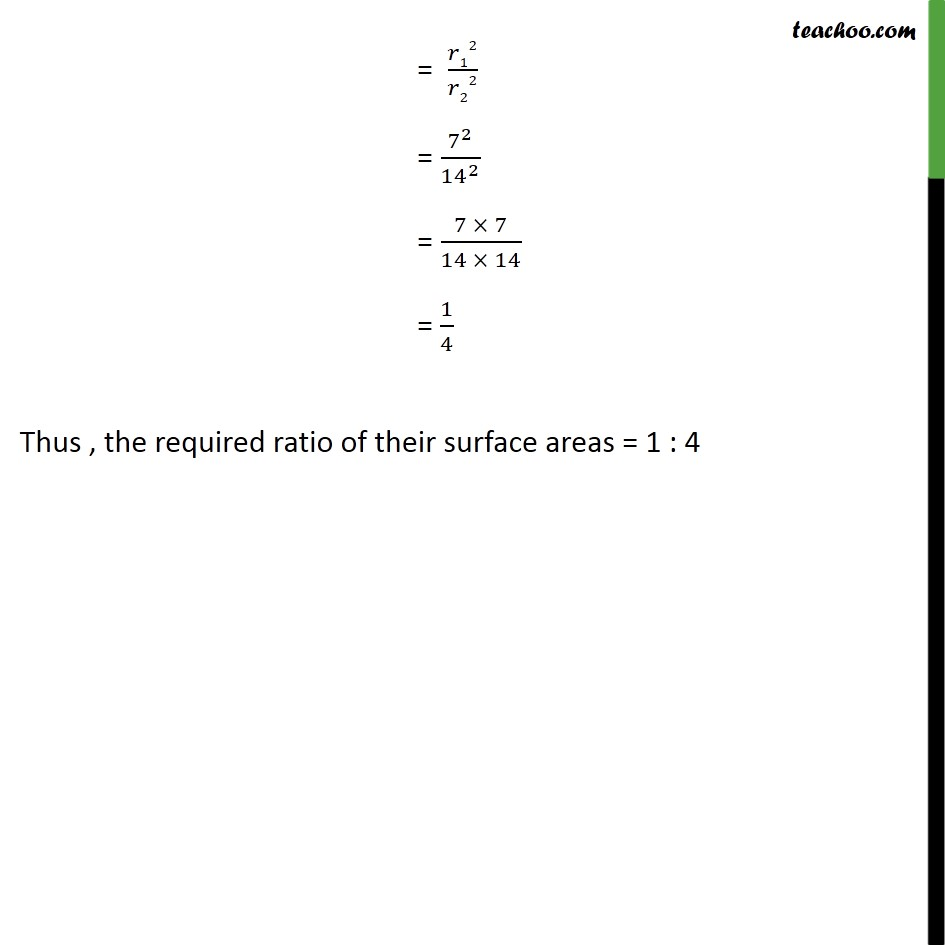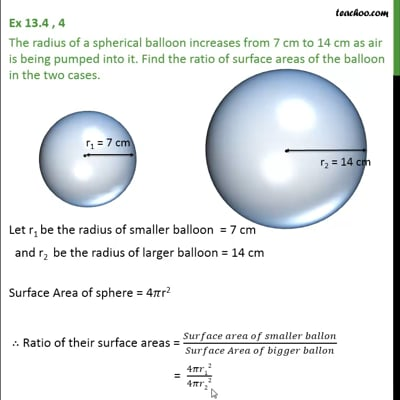Ex 13.4

Chapter 13 Class 9 Surface Areas and Volumes
Serial order wiseThis video is only available for Teachoo black users

Maths Crash Course - Live lectures + all videos + Real time Doubt solving!

### Transcript

Ex 13.4 , 4 The radius of a spherical balloon increases from 7 cm to 14 cm as air is being pumped into it. Find the ratio of surface areas of the balloon in the two cases. Let r1 be the radius of smaller balloon = 7 cm and r2 be the radius of larger balloon = 14 cm Surface Area of sphere = 4 r2 Ratio of their surface areas = ( )/( ) = 4 12/4 22 = 12/ 22 = 7^2/ 14 ^2 = (7 7)/(14 14) = 1/4 Thus , the required ratio of their surface areas = 1 : 4